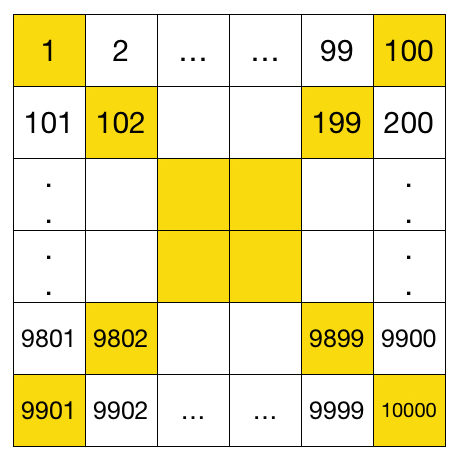# Diagonal Sum

Algebra Level 3

Integers from 1 to 10000 are written orderly in a grid of $100\times 100$, as shown in the table below.

What is the sum of the numbers in the two diagonals highlighted in yellow?×